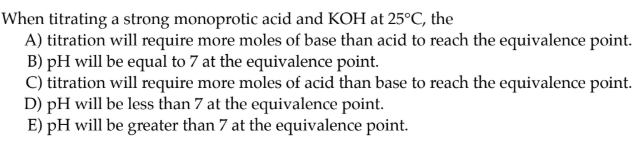# When titrating a strong monoprotic acid and KOH at 25°C, the A) titration will require more moles of base than acid to reach the equivalence point. B) pH will be equal to 7 at the equivalence point. C) titration will require more moles of acid than base to reach the equivalence point. D) pH will be less than 7 at the equivalence point. E) pH will be greater than 7 at the equivalence point.